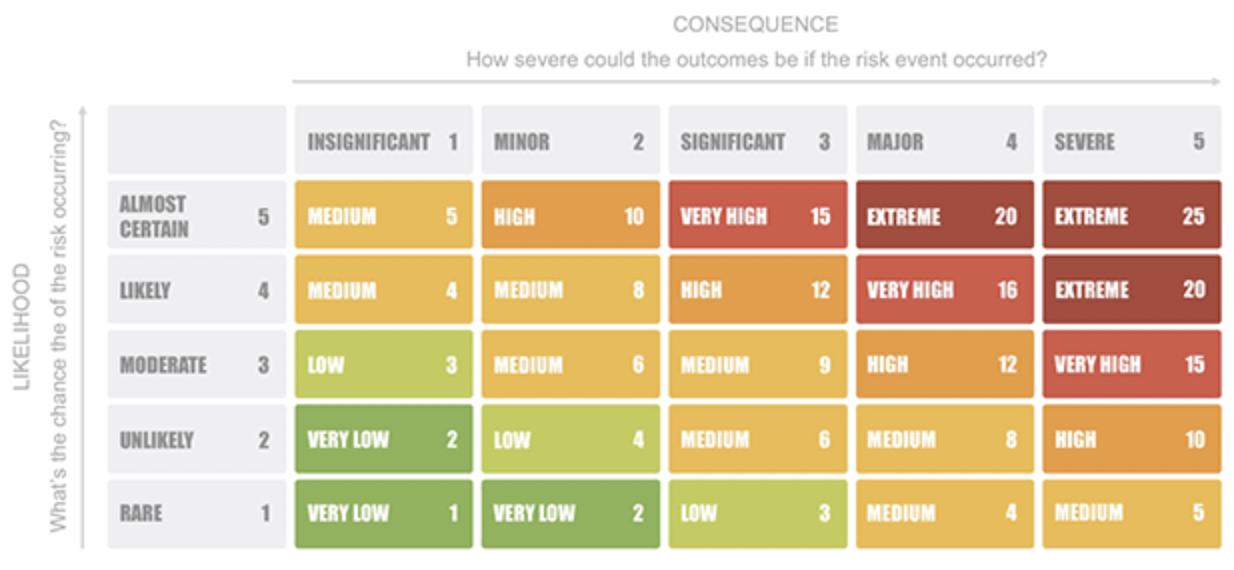Title Page

• Site conducted

• Activity Assessed

• Issue number

• Purpose of new issue

• Add detailed description of review

• Location
• Assessment Date

• Review Date

• Assessor (name)

• Assisted by (names)

Hazard List

• What is the source of Hazard?

• Who is exposed?

• What is the type of injury that could occur?

• What are the existing controls?

• How much is the likelihood of occurrence of hazard

• What could be the severity of Injury/ ?isease

• Use the Risk Matrix to determine the risk rating for this hazard• What is the risk rating for this hazard?

• Use the Risk Matrix to determine the risk rating for this hazard• What is the risk rating for this hazard?

• Use the Risk Matrix to determine the risk rating for this hazard• What is the risk rating for this hazard?

• Use the Risk Matrix to determine the risk rating for this hazard• What is the risk rating for this hazard?

• Use the Risk Matrix to determine the risk rating for this hazard• What is the risk rating for this hazard?

• please first go and complete the risk assessment for COSHH/ handling hazardous materials and ensure you have a current safety data sheet available

• Source of Hazard

• Who is exposed

• What is the type of injury that could occur?

• Existing Controls

• How much is the likelihood of occurrence of hazard

• What could be the severity of Injury/ Disease

• Use the Risk Matrix to determine the risk rating for this hazard• What is the risk rating for this hazard?

• Use the Risk Matrix to determine the risk rating for this hazard• What is the risk rating for this hazard?

• Use the Risk Matrix to determine the risk rating for this hazard• What is the risk rating for this hazard?

• Use the Risk Matrix to determine the risk rating for this hazard• What is the risk rating for this hazard?

• Use the Risk Matrix to determine the risk rating for this hazard• What is the risk rating for this hazard?

• please first complete the risk assessment for Manual handling

• Source of Hazard

• Who is exposed

• What is the type of injury that could occur?

• Existing Controls

• How much is the likelihood of occurrence of hazard

• What could be the severity of Injury/ Disease

• Use the Risk Matrix to determine the risk rating for this hazard• What is the risk rating for this hazard?

• Use the Risk Matrix to determine the risk rating for this hazard• What is the risk rating for this hazard?

• Use the Risk Matrix to determine the risk rating for this hazard• What is the risk rating for this hazard?

• Use the Risk Matrix to determine the risk rating for this hazard• What is the risk rating for this hazard?

• Use the Risk Matrix to determine the risk rating for this hazard• What is the risk rating for this hazard?

• please first go and complete the risk assessment for using display screen equipment (DSE)

• Source of Hazard

• Who is exposed

• What is the type of injury that could occur?

• Existing Controls

• How much is the likelihood of occurrence of hazard

• What could be the severity of Injury/ Disease

• Use the Risk Matrix to determine the risk rating for this hazard• What is the risk rating for this hazard?

• Use the Risk Matrix to determine the risk rating for this hazard• What is the risk rating for this hazard?

• Use the Risk Matrix to determine the risk rating for this hazard• What is the risk rating for this hazard?

• Use the Risk Matrix to determine the risk rating for this hazard• What is the risk rating for this hazard?

• Use the Risk Matrix to determine the risk rating for this hazard• What is the risk rating for this hazard?

• Other Hazard

• Source of Hazard

• Who is exposed

• What is the type of injury that could occur?

• Existing Controls

• How much is the likelihood of occurrence of hazard

• What could be the severity of Injury/ Disease

• Use the Risk Matrix to determine the risk rating for this hazard• What is the risk rating for this hazard?

• Use the Risk Matrix to determine the risk rating for this hazard• What is the risk rating for this hazard?

• Use the Risk Matrix to determine the risk rating for this hazard• What is the risk rating for this hazard?

• Use the Risk Matrix to determine the risk rating for this hazard• What is the risk rating for this hazard?

• Use the Risk Matrix to determine the risk rating for this hazard• What is the risk rating for this hazard?

Control Measures and Personal Protective Equipment Required

• Are machinery protection devices (guards, interlocks) used as a control measure for this task?

• Is there a specific system of work (e.g. SSW, JSA, Work at Height Permit) required for this task?

• Is Hearing Protection required for this task?

• Is Eye Protection required for this task?

• Is Respiratory Protection required for this task?

• Is Hand Protection required for this task?

• Is protective coveralls/ clothing required for this task?

• Is Hi Vis Clothing required for this task?

• Is safety footwear required for this task?

• Is there other personal protective equipment required for this task?